# AP Chemistry : Yield and Error

## Example Questions

### Example Question #1 : Yield And Error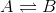3 mols ofare placed in a reactor and react to formas in the above reaction. At equilibrium, there are 1.75 mols of.

What is the percent conversion of?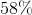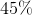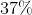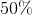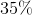Explanation:

To find the percent conversion, use the following equation: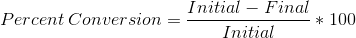Initially there are 3 mols of. We know that every 1 mol ofthat is consumed in the reaction yields 1 mol of. Therefore, the final amount ofin mols is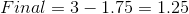Plugging this into the above equation gives a conversion rate of 58%.

### Example Question #2 : Yield And Error

You run an experiment in order to empirically find the gas constant,.  You find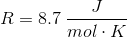You know from the literature that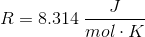What is the percent error in your experimental value of?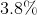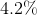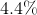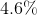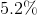Explanation:

To find the percent error in yourvalue, you can use the following equation: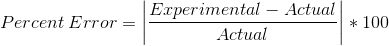Doing this, we find the percent error to be 4.6%.

### Example Question #3 : Yield And Error

Suppose that a chemist working in a lab is trying to synthesize caffeine. In doing so, he predicts that he can produce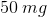of caffeine. After the chemist finishes synthesizing the caffeine, he weighs it only to find out that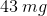are produced. What is the percent yield of caffeine in this synthesis process?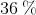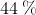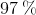Explanation:

When chemists synthesize compounds in the lab, a theoretical yield can be calculated by using knowledge of the full, balanced chemical equation, as well as the starting amounts of all reagents including the limiting reagent. However, due to a variety of factors, the actual yield obtained at the end of the experiment is nearly always going to be lower than the theoretical yield. Some of the things that can cause this are experimental error and side reactions, among others. Thus, chemists usually take the ratio of actual yield to theoretical yield to give the percent yield.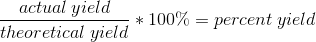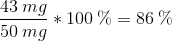This means that the overall process was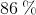efficient.